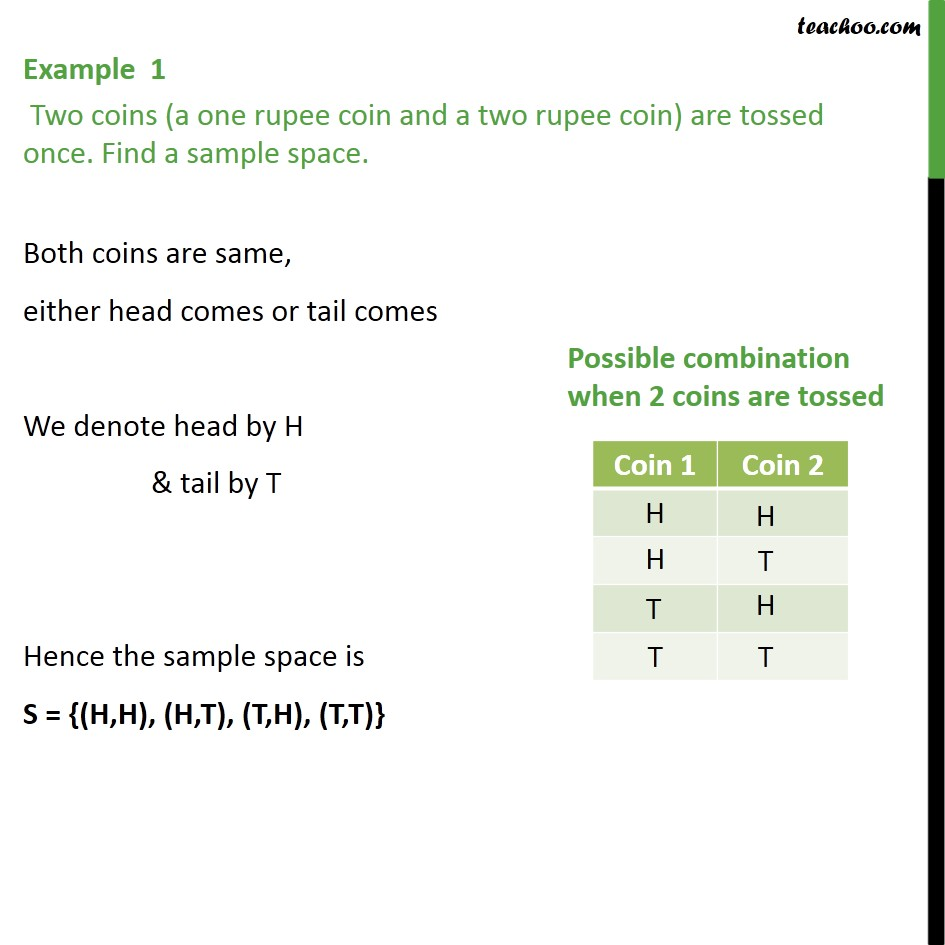Examples

Chapter 16 Class 11 Probability
Serial order wiseMaths Crash Course - Live lectures + all videos + Real time Doubt solving!

### Transcript

Example 1 Two coins (a one rupee coin and a two rupee coin) are tossed once. Find a sample space. Both coins are same, either head comes or tail comes We denote head by H & tail by T Hence the sample space is S = {(H,H), (H,T), (T,H), (T,T)}